# Appendix D:

The purpose of this appendix is to develop the matrix coefficients (E, F, G) and the forcing function (R) from Equation (26). In the text that follows, the contributions to E, F, G and R are given on a term-by-term basis. The final values are determined by summing the individual contributions.

Equations (B7), (B11), and (B17) are used to approximate the spatial derivative describing advective transport. These equations contribute to the matrix coefficients as detailed below. Due to boundary conditions, the contributions depend on the type of segment for which Equation (26) is developed.

### Interior Segments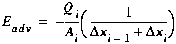(D1)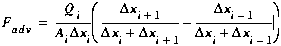(D2)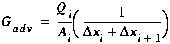(D3)

### First Segment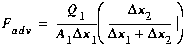(D4)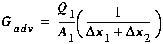(D5)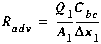(D6)

### Last Segment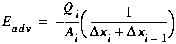(D7)(D8)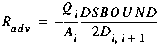(D9)

## D2.0 Dispersion

As with the advection term, the finite difference equations given in Appendix B (Equations (B9), (B14) and (B18)) are used to determine the contributions to the matrix coefficients.

### Interior Segments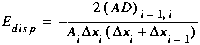(D10)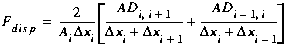(D11)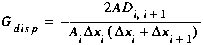(D12)

### First Segment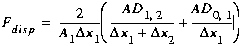(D13)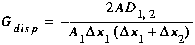(D14)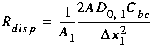(D15)

### Last Segment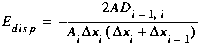(D16)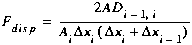(D17)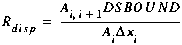(D18)

## D3.0 Lateral Inflow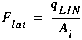(D19)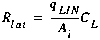(D20)

## D4.0 Transient Storage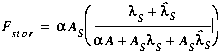(D21)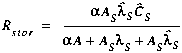(D22)

## D5.0 Decay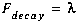(D23)

## D6.0 Matrix Coefficients

The matrix coefficients (E, F, G) and the forcing function (R) from Equation (26) may now be developed by summing the contributions given in Sections D1.0 through D5.0;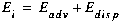(D24)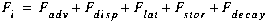(D25)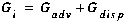(D26)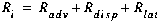(D27)